# GATE CS 2021 | Set 2

• Last Updated : 18 Oct, 2021

 Question 1
Gauri said that she can play the keyboard __________ her sister.
 A as well as B as better as C as nicest as D as worse as
GATE CS 2021 | Set 2    English
Discuss it

Question 1 Explanation:
‘worse’, ‘better’ are comparative words which need the word ‘than’ for comparison. Out of the remaining two options ‘as well as’ is the correct answer. Correct Option (A).
 Question 2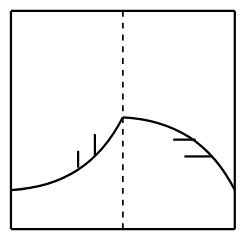A transparent square sheet shown above is folded along the dotted line. The folded sheet will look like ___________.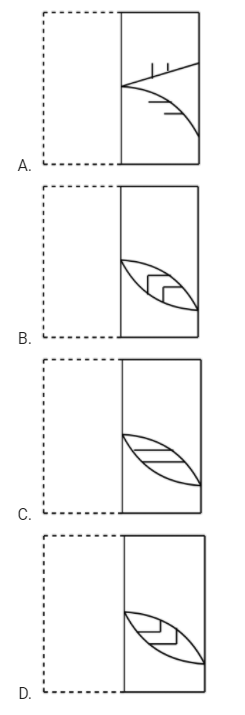A A B B C C D D
GATE CS 2021 | Set 2    General Aptitude
Discuss it

Question 2 Explanation:
When the sheet is folded, the vertical lines of the sheet will be above than the horizontal lines. So option (B) is true.
 Question 3
If θ is the angle, in degrees, between the longest diagonal of the cube and any one of the edges of the cube, then, cosθ =
 A 1/2 B 1/√3 C 1/√2 D √3/2
GATE CS 2021 | Set 2    General Aptitude    50 Aptitude & Reasoning MCQs with Answers
Discuss it

Question 3 Explanation:
We may assume the length of a side of the cube is 1. Then the diagonal is given by the vector v = <1, 1, 1>. The 3 sides are given by the vectors i = <1, 0, 0>, j = <0, 1, 0> and k = <0, 0, 1>. Each gives the same angle.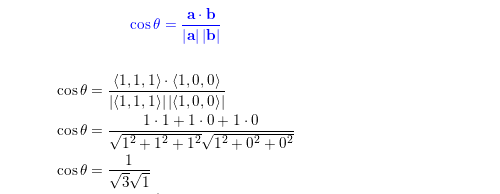Alternative Way : cosθ = Base / Hypotenuse = a3√a=13√ Length of diagonal of a side = √(a2 + a2) = √(2a) Length of the longest diagonal = √( a2 +(√(2a))2 ) = √(3)a = Hypotenuse Therefore, cosθ = Base / Hypotenuse = a/(√(3)a) = 1/√3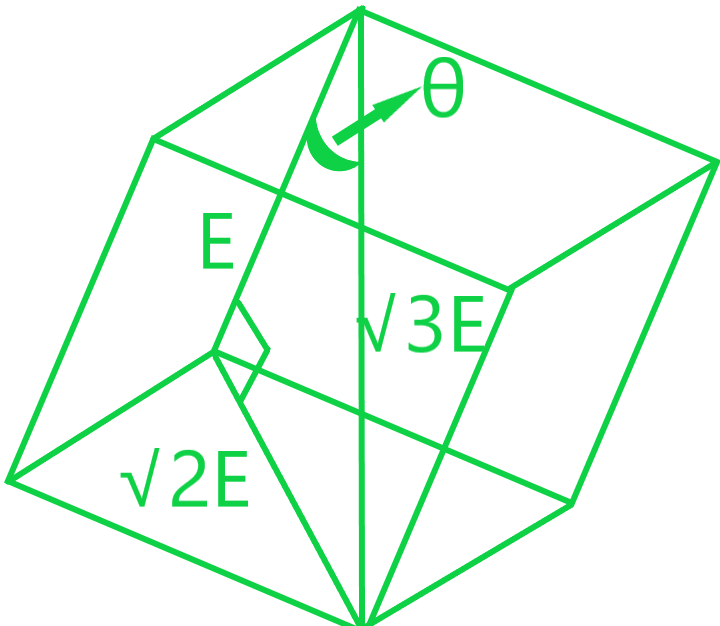Question 4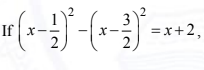then the value of x is:
 A 2 B 4 C 6 D 8
GATE CS 2021 | Set 2    General Aptitude
Discuss it

Question 4 Explanation:
Apply formula a2 - b2 = (a+b)*(a-b) You will get x = 4.
 Question 5
`Pen : Write :: Knife : _______ `
Which one of the following options maintains a similar logical relation in the above?
 A Vegetables B Sharp C Cut D Blunt
GATE CS 2021 | Set 2    English
Discuss it

Question 5 Explanation:
A pen is used to write. Similarly, a knife is used to cut. Correct Option (C)
 Question 6
Listening to music during exercise improves performance and reduces discomfort. Scientists researched whether listening to music while studying can help students learn better and the results were inconclusive. Students who needed external stimulation for studying fared worse while students who did not need any external stimulation benefited from music. Which one of the following statements is the CORRECT inference of the above passage?
 A Listening to music has no effect on learning and a positive effect on physical exercise B Listening to music has a clear positive effect both in physical exercise and on learning C Listening to music has a clear positive effect on physical exercise. Music has a positive effect on learning only in some students D Listening to music has a clear positive effect on learning in all students. Music has a positive effect only in some students who exercise
GATE CS 2021 | Set 2    English
Discuss it

Question 6 Explanation:
From the first statement “Listening to music during exercise improves exercise performance and reduces discomfort. “ It is clear that listening to music has a positive effect on physical exercise. From the statement “Scientists researched whether listening to music while studying can help students learn better and the results were inconclusive. “ it is clear that only on some students music has a positive effect.
 Question 7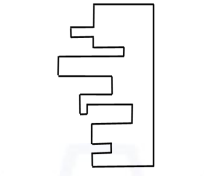A jigsaw puzzle has 2 pieces. One of the pieces is shown above. Which one of the given options for the missing piece when assembled will form a rectangle? The piece can be moved, rotated or flipped to assemble with the above piece.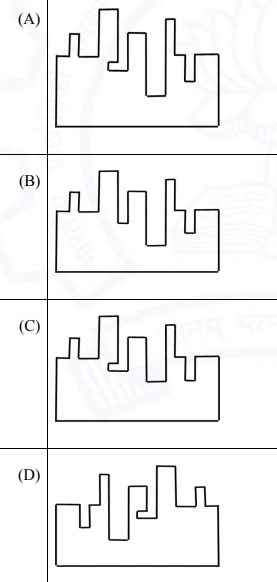A A B B C C D D
GATE CS 2021 | Set 2    General Aptitude
Discuss it

Question 7 Explanation:
When we join the missing piece, A rectangle should be formed. If we carefully notice the L shape part of the shape, we can easily eliminate option B and option D. Now if we notice option A and option C, option A is perfectly matching to complete the shape.
 Question 8
The number of students in three classes is in the ratio 3:13:6. If 18 students are added to each class, the ratio changes to 15:35:21. The total number of students in all the three classes in the beginning was:
 A 22 B 66 C 88 D 110
GATE CS 2021 | Set 2    Probability    Set Theory & Algebra
Discuss it

Question 8 Explanation:
According to given conditions, 3x+18 : 13x+18 : 6x+18 = 15 : 35 : 21 On solving, (3x+18) / (13x+18) = 15/35 105x + 630 = 195x + 270 195x - 105x = 630 - 270 90x = 360 x = 360/90 = 4 So total number of students in these three classes, 3*4 = 12 13*4 = 52 6*4 = 24 In total, 88 students are there.
 Question 9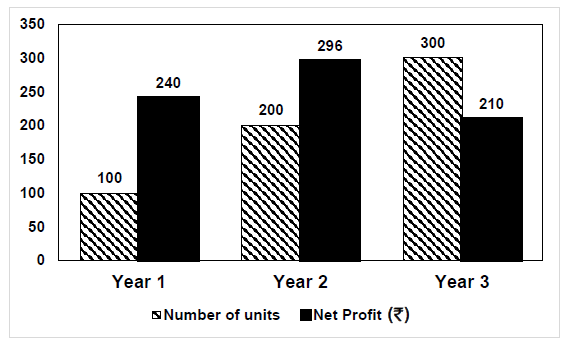The number of units of a product sold in three different years and the respective net profits are presented in the figure above. The cost/unit in Year 3 was Re. 1, which was half the cost/unit in Year 2. The cost/unit in Year 3 was one-third of the cost/unit in Year 1. Taxes were paid on the selling price at 10%, 13%, and 15% respectively for the three years. Net profit is calculated as the difference between the selling price and the sum of cost and taxes paid in that year. The ratio of the selling price in Year 2 to the selling price in Year 3 is _________.
 A 4:3 B 1:1 C 3:4 D 1:2
GATE CS 2021 | Set 2    General Aptitude    Ratio and Proportion
Discuss it

Question 9 Explanation:

From the above graph we obtained following information-

```Year    Cost/Unit   Cost Price    Number of Units
1             3              300             100
2             2              400             200
3             1              300             300```

Let selling price of year 2 = SP2 and Selling price of year3 = SP3

Given that-

```Taxes for year 2 = 13% of SP2 = 0.13SP2
Taxes for year 3 = 15% of SP3 = 0.15SP3
Cost per unit of year 3 = 1
(Cost per unit of year 2)/2 = 1
So now Cost / unit of year 2 = 2*1 = 2```

Net profit formula now = Selling Price - (Cost Price + (Tax% x selling price )

For year 2

```296 = SP2- (200*2 + 0.13*SP2)
SP2 = 696*100/87
SP2 = 800```

For year 3

```210 = SP3 - (300*1+0.15*SP3)
SP3 = 510*100/85
SP3 = 600```

Required Ratio 800/600= 4/3 (Correct Option A)

 Question 10
Six students P, Q, R, S, T and U, with distinct heights, compare their heights and make the following observations.
• Observation I: S is taller than R.
• Observation II: Q is the shortest of all.
• Observation III: U is taller than only one student.
• Observation IV: T is taller than S but is not the tallest.
The number of students that are taller than R is the same as the number of students shorter than ____________.
 A T B R C S D P
GATE CS 2021 | Set 2    General Aptitude
Discuss it

Question 10 Explanation:
From observations II and III, we can say that Q is the shortest. U is the second shortest.
` __ __ __ __ U Q `
From observations I and IV, we can say that T is the second tallest. S is the third tallest. R is the fourth tallest. Thus, P is the tallest. Therefore, the final arrangement is as follows,
`P T S R U Q `
Three students are taller than R, hence three students are shorter than S.
There are 65 questions to complete.
My Personal Notes arrow_drop_up